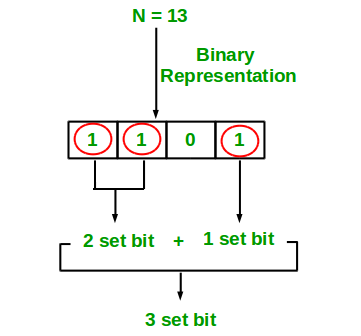# C/C++ Program to Count set bits in an integer

Write an efficient program to count number of 1s in binary representation of an integer.

Examples :

```Input : n = 6
Output : 2
Binary representation of 6 is 110 and has 2 set bits

Input : n = 13
Output : 3
Binary representation of 11 is 1101 and has 3 set bits
```## Recommended: Please solve it on “PRACTICE ” first, before moving on to the solution.

1. Simple Method Loop through all bits in an integer, check if a bit is set and if it is then increment the set bit count. See below program.

## C

 `#include ` ` `  `/* Function to get no of set bits in binary ` `   ``representation of positive integer n */` `unsigned ``int` `countSetBits(unsigned ``int` `n) ` `{ ` `    ``unsigned ``int` `count = 0; ` `    ``while` `(n) { ` `        ``count += n & 1; ` `        ``n >>= 1; ` `    ``} ` `    ``return` `count; ` `} ` ` `  `/* Program to test function countSetBits */` `int` `main() ` `{ ` `    ``int` `i = 9; ` `    ``printf``(``"%d"``, countSetBits(i)); ` `    ``return` `0; ` `} `

Output:

```2
```

Recursive Approach :

## C++

 `// Cpp implementation of recursive ` `// approach to find the number ` `// of set bits in binary representation ` `// of positive integer n ` `#include ` `using` `namespace` `std; ` ` `  `// recursive function to count set bits ` `int` `countSetBits(``int` `n) ` `{ ` `    ``// base case ` `    ``if` `(n == 0) ` `        ``return` `0; ` ` `  `    ``else` ` `  `        ``// if last bit set add 1 else add 0 ` `        ``return` `(n & 1) + countSetBits(n >> 1); ` `} ` ` `  `// driver code ` `int` `main() ` `{ ` `    ``// get value from user ` `    ``int` `n = 9; ` ` `  `    ``// function calling ` `    ``cout << countSetBits(n); ` ` `  `    ``return` `0; ` `} ` ` `  `// This code is contributed by Raj. `

Output:

```2
```

Please refer complete article on Count set bits in an integer for more details!

My Personal Notes arrow_drop_up
Article Tags :

Be the First to upvote.

Please write to us at contribute@geeksforgeeks.org to report any issue with the above content.